# KR101278951B1 - Mixed type frequency compensating circuit, control circuit, dc-dc converter and method of controlling the same - Google Patents

Mixed type frequency compensating circuit, control circuit, dc-dc converter and method of controlling the same Download PDF

## Info

Publication number
KR101278951B1
KR101278951B1 KR1020060046490A KR20060046490A KR101278951B1 KR 101278951 B1 KR101278951 B1 KR 101278951B1 KR 1020060046490 A KR1020060046490 A KR 1020060046490A KR 20060046490 A KR20060046490 A KR 20060046490A KR 101278951 B1 KR101278951 B1 KR 101278951B1
Authority
KR
South Korea
Prior art keywords
voltage
compensation circuit
frequency compensation
circuit
voltage signal
Prior art date
Application number
KR1020060046490A
Other languages
Korean (ko)
Other versions
KR20070113520A (en
Inventor
정상화
김동희
이경구
이동훈
Original Assignee
페어차일드코리아반도체 주식회사
Priority date (The priority date is an assumption and is not a legal conclusion. Google has not performed a legal analysis and makes no representation as to the accuracy of the date listed.)
Filing date
Publication date
Application filed by 페어차일드코리아반도체 주식회사 filed Critical 페어차일드코리아반도체 주식회사
Priority to KR1020060046490A priority Critical patent/KR101278951B1/en
Publication of KR20070113520A publication Critical patent/KR20070113520A/en
Application granted granted Critical
Publication of KR101278951B1 publication Critical patent/KR101278951B1/en

• 230000001276 controlling effects Effects 0.000 title description 2
• 239000003990 capacitor Substances 0.000 claims abstract description 56
• 239000004065 semiconductor Substances 0.000 claims abstract description 29
• 230000000051 modifying Effects 0.000 claims description 14

## Images

•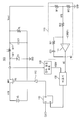••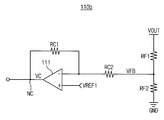•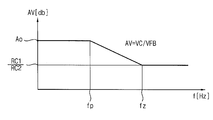•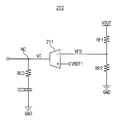•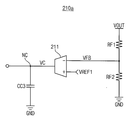••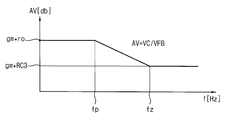•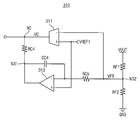••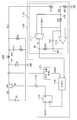•••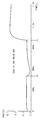•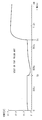## Classifications

• HELECTRICITY
• H02GENERATION; CONVERSION OR DISTRIBUTION OF ELECTRIC POWER
• H02MAPPARATUS FOR CONVERSION BETWEEN AC AND AC, BETWEEN AC AND DC, OR BETWEEN DC AND DC, AND FOR USE WITH MAINS OR SIMILAR POWER SUPPLY SYSTEMS; CONVERSION OF DC OR AC INPUT POWER INTO SURGE OUTPUT POWER; CONTROL OR REGULATION THEREOF
• H02M3/00Conversion of dc power input into dc power output
• H02M3/02Conversion of dc power input into dc power output without intermediate conversion into ac
• H02M3/04Conversion of dc power input into dc power output without intermediate conversion into ac by static converters
• H02M3/10Conversion of dc power input into dc power output without intermediate conversion into ac by static converters using discharge tubes with control electrode or semiconductor devices with control electrode
• H02M3/145Conversion of dc power input into dc power output without intermediate conversion into ac by static converters using discharge tubes with control electrode or semiconductor devices with control electrode using devices of a triode or transistor type requiring continuous application of a control signal
• H02M3/155Conversion of dc power input into dc power output without intermediate conversion into ac by static converters using discharge tubes with control electrode or semiconductor devices with control electrode using devices of a triode or transistor type requiring continuous application of a control signal using semiconductor devices only
• H02M3/156Conversion of dc power input into dc power output without intermediate conversion into ac by static converters using discharge tubes with control electrode or semiconductor devices with control electrode using devices of a triode or transistor type requiring continuous application of a control signal using semiconductor devices only with automatic control of output voltage or current, e.g. switching regulators

## Abstract

A mixed frequency compensation circuit having a partial circuit of a voltage amplifier type frequency compensation circuit and a partial circuit of a transconductance amplifier type frequency compensation circuit is disclosed. The mixed frequency compensation circuit includes an integral component sub circuit of the voltage amplifier type frequency compensation circuit and a proportional component sub circuit of the transconductance amplifier type frequency compensation circuit. The integral component subcircuit of the voltage amplifier type frequency compensation circuit amplifies the input voltage signal in voltage mode and compensates the frequency characteristic, and the proportional component subcircuit of the transconductance amplifier type frequency compensation circuit amplifies the input voltage signal in current mode. Compensate for frequency characteristics. Therefore, the capacitance of the frequency compensating capacitor included in the mixed frequency compensating circuit is small and the resistance value of the frequency compensating resistor is small, so that it is easy to be incorporated into a semiconductor integrated circuit.

## Description

Mixed frequency compensation circuit, control circuit, DC-DC converter and control method thereof {MIXED TYPE FREQUENCY COMPENSATING CIRCUIT, CONTROL CIRCUIT, DC-DC CONVERTER AND METHOD OF CONTROLLING THE SAME}

1 is a circuit diagram showing a conventional DC-DC converter having a voltage amplifier type frequency compensation circuit.

FIG. 2A is a circuit diagram illustrating an integrated component subcircuit of a voltage amplifier type frequency compensation circuit included in the DC-DC converter of FIG. 1.

FIG. 2B is a circuit diagram illustrating a proportional component subcircuit of the voltage amplifier type frequency compensation circuit included in the DC-DC converter of FIG. 1.

FIG. 2C is a graph illustrating voltage gain versus frequency of the frequency compensation circuit included in the DC-DC converter of FIG. 1.

3 is a circuit diagram showing a transconductance amplifier type frequency compensation circuit.

FIG. 4A is a circuit diagram showing an integrated component subcircuit of the transconductance amplifier type frequency compensation circuit shown in FIG.

4B is a circuit diagram illustrating a proportional component subcircuit of the transconductance amplifier type frequency compensation circuit shown in FIG. 3.

FIG. 5 is a graph showing voltage gain vs. frequency of the frequency compensation circuit shown in FIG.

6 is a circuit diagram illustrating a mixed type frequency compensation circuit according to an embodiment of the present invention.

7 is a graph illustrating a voltage gain of the mixed frequency compensation circuit of FIG. 6.

FIG. 8 is a circuit diagram illustrating an embodiment of a DC-DC converter including the mixed frequency compensation circuit of FIG. 6.

9A and 9B are graphs showing waveforms of output signals of the frequency compensation circuit according to the prior art and the present invention, respectively.

10A and 10B are graphs showing waveforms of a DC-DC converter output signal according to the related art and the present invention, respectively.

Description of the Related Art 

100, 1000: DC-DC converter

110: voltage amplifier type frequency compensation circuit

111, 1113: operational amplifier

120, 1120: current detection circuit

130, 1130: comparator

140, 1140: RS-Flip-Flop

150, 1150: Buffer

210: transconductance amplifier type frequency compensation circuit

211, 1111: transconductance amplifier

1100: control circuit

The present invention relates to a frequency compensation circuit, and more particularly to a mixed frequency compensation circuit.

1 is a circuit diagram showing a conventional DC-DC converter having a voltage amplifier type frequency compensation circuit. The DC-DC converter shown in FIG. 1 is a buck type DC-DC converter that generates a DC output voltage VOUT lower than the DC input voltage VIN.

Referring to FIG. 1, the DC-DC converter 100 includes a DC voltage source VS, a switching transistor MN1, a diode D1, an inductor L1, a capacitor C1, a resistor RL, and a frequency compensation circuit. 110, a current detection circuit 120, a comparator 130, an RS-flip-flop 140, and a buffer 1150.

The switching transistor MN1 is driven in response to the gate driving signal VG and provides an input voltage VIN to the node N11. Diode D1 has a cathode coupled to node N11 and an anode coupled to ground GND. Inductor L1 is coupled between node N11 and node N12. Capacitor C1 is coupled between node N12 and ground GND. Resistor RL is coupled between node N12 and ground GND as a load resistor.

The frequency compensating circuit 110, the current detecting circuit 120, the comparator 130, the RS-flip flop 140, and the buffer 150 may include a switching transistor MN1 included in the DC-DC converter 100. A control circuit for driving is constituted. The control circuit generates a gate driving signal VG and provides the gate driving signal VG to the gate of the switching transistor MN1.

The frequency compensation circuit 110 includes an operational amplifier 111, feedback resistors RF1 and RF2, resistors RC1 and RC2, and a capacitor CC1. The frequency compensation circuit 110 generates a compensation voltage signal VC by amplifying the feedback voltage signal VFB as a voltage amplifier type frequency compensation circuit.

The current detection circuit 120 detects a current flowing through the switching transistor MN1 and generates a detection voltage signal VSEN1. The comparator 130 compares the voltage level of the output signal VC of the frequency compensation circuit 110 with the voltage level of the detection voltage signal VSEN1. The output signal of the comparator 130 is input to the reset terminal of the RS flip-flop 140. The clock signal CLK is applied to the set terminal of the RS flip-flop 1140. The buffer 150 buffers the output signal of the RS flip-flop 140 to generate the gate driving signal VG. The gate driving signal VG is applied to the gate of the switching transistor MN1.

The frequency compensation circuit 110 included in the DC-DC converter of FIG. 1 is a voltage amplifier type frequency compensation circuit. The capacitor CC1 inserted for frequency compensation in the voltage amplifier type frequency compensation circuit 110 included in FIG. 1 has a small capacitance, but the resistor RC1 has a large resistance. For example, CC1 may have a capacitance of 10 pF, and RC1 may have a resistance value of 15.9 MΩ. A capacitor having a capacitance of 10 PF is easy to embed in a semiconductor integrated circuit, but a resistor having a resistance value of 15.9 MΩ occupies a large chip area of the semiconductor integrated circuit and thus is difficult to embed in a semiconductor integrated circuit.

The voltage amplifier type frequency compensation circuit 110 may be viewed as a circuit in which an integral component subcircuit 110a and a proportional component subcircuit 110b illustrated in FIG. 2A are superposed.

In the integrated component subcircuit 110a of FIG. 2A, only the capacitor CC1 is coupled between the output terminal of the operational amplifier 111 and the inverting input terminal, and the resistor RC1 is not coupled. In the proportional component subcircuit 110b of FIG. 2B, only the resistor RC1 is coupled between the output terminal of the operational amplifier 111 and the inverting input terminal, and the capacitor CC1 is not coupled.

FIG. 2C is a graph illustrating voltage gain versus frequency of the frequency compensation circuit 110 included in the DC-DC converter of FIG. 1. Referring to FIG. 2C, the voltage gain AV maintains Ao and starts to decrease at the pole frequency fp to maintain the value of RC1 / RC2 from zero frequency fz. The pole frequency fp is fp = 1 / {2π (Ao * RC2 * CC1)} and the zero frequency fz is fz = 1 / {2π (RC1 * CC1)}. Here, Ao represents the open loop gain of the operational amplifier 111.

3 is a circuit diagram illustrating a transconductance amplifier type frequency compensation circuit 210. The transconductance amplifier type frequency compensation circuit 210 of FIG. 3 may be used instead of the voltage amplifier type frequency compensation circuit 110 included in the DC-DC converter of FIG. 1.

The frequency compensation circuit 210 includes a transconductance amplifier 211, feedback resistors RF1 and RF2, a resistor RC3, and a capacitor CC3. The frequency compensation circuit 210 is a transconductance amplifier type frequency compensation circuit and amplifies the feedback voltage signal VFB to generate the compensation voltage signal VC.

The resistor RC3 inserted for the frequency compensation in the transconductance amplifier type frequency compensation circuit 210 of FIG. 3 has a small value, but the capacitor CC3 has a large value of capacitance. For example, RC1 may have a resistance value of 191 kΩ and CC1 may have a capacitance of 830 pF. A resistor having a resistance value of 191 kΩ is easy to embed in a semiconductor integrated circuit, but a capacitor having a capacitance of 830 pF occupies a large chip area of the semiconductor integrated circuit and thus is difficult to embed in a semiconductor integrated circuit.

The transconductance amplifier type frequency compensation circuit 210 may be viewed as a circuit in which the integral component subcircuit 210a and the proportional component subcircuit 210b shown in FIG. 4A are superposed. .

In the integrated component subcircuit 210a of FIG. 4A, only the capacitor CC3 is coupled between the output terminal of the transconductance amplifier 211 and the ground, and the resistor RC3 is not coupled. In the proportional component subcircuit 210b of FIG. 4B, only the resistor RC3 is coupled between the output terminal of the transconductance amplifier 211 and the ground, and the capacitor CC3 is not coupled.

FIG. 5 is a graph showing voltage gain versus frequency of the frequency compensation circuit 210 shown in FIG. Referring to FIG. 5, the voltage gain AV maintains the value of gm * ro and then decreases at the pole frequency fp and stops decreasing at the zero frequency fz and maintains the value of gm * RC3. The pole frequency fp is fp = 1 / {2π (ro * CC3)} and the zero frequency fz is fz = 1 / {2π (RC3 * CC3)}. Here, gm represents the transconductance of the transconductance amplifier 211 and ro represents the output resistance of the transconductance amplifier 211.

In the transconductance amplifier type frequency compensation circuit 210 of Figure 3, the value of the transconductance (gm) of the transconductance amplifier (211) gm = 200 * 10 -6 A / V, RC3 = 191 kΩ, CC3 = 830 pF In this case, the zero frequency fz may be represented by fz = 1 / {2π (RC3 * CC3)} and has a value of about 1 kHz. The voltage gain (AV) can be expressed as AV = gm * RC3, which is about 31.6 dB (= 20 log 38).

As described above, the resistor RC1 inserted for frequency compensation in the voltage amplifier type frequency compensation circuit 110 included in the DC-DC converter of FIG. 1 has a very large resistance value, for example, a resistance value of 15.9 MΩ. Therefore, it is difficult to embed the resistor RC1 in the semiconductor integrated circuit. In addition, since the capacitor CC3 inserted for frequency compensation in the transconductance amplifier type frequency compensation circuit 210 of FIG. 3 has a very large capacitance, for example, a capacitance of 830 pF, the capacitor CC3 is a semiconductor integrated circuit. Difficult to build in.

Accordingly, there is a need for a frequency compensation circuit capable of embedding a capacitor and a resistor inserted into the frequency compensation circuit for the frequency compensation in a semiconductor integrated circuit.

An object of the present invention is to provide a mixed frequency compensation circuit having a frequency compensation capacitor and a resistor that can be embedded in a semiconductor integrated circuit.

Another object of the present invention is to provide a control circuit having a mixed frequency compensation circuit.

Still another object of the present invention is to provide a DC-DC converter having a mixed frequency compensation circuit.

It is still another object of the present invention to provide a control method of a mixed frequency compensation circuit having a frequency compensation capacitor and a resistor that can be embedded in a semiconductor integrated circuit.

Still another object of the present invention is to provide a control method of a DC-DC converter having a mixed frequency compensation circuit.

In order to achieve the above object, a mixed frequency compensation circuit according to an embodiment of the present invention includes an integral component sub circuit of a voltage amplifier type frequency compensation circuit and a proportional component sub circuit of a transconductance amplifier type frequency compensation circuit.

The integral component subcircuit of the voltage amplifier type frequency compensation circuit amplifies the input voltage signal in voltage mode and compensates the frequency characteristic, and the proportional component subcircuit of the transconductance amplifier type frequency compensation circuit amplifies the input voltage signal in current mode. Compensate for frequency characteristics.

According to one embodiment of the invention, the mixed frequency compensation circuit includes a transconductance amplifier, a first resistor, a second resistor, an operational amplifier, and a first capacitor.

The transconductance amplifier has a first input terminal to which the input voltage signal is applied and a second input terminal to which a reference voltage is applied, and amplifies the input voltage signal in a current mode and outputs it to an output node. A first resistor is coupled between the output node and the first node, and the second resistor has a first terminal to which the input voltage signal is applied. The operational amplifier amplifies the input voltage signal in a voltage mode with a first input terminal coupled to a second terminal of the second resistor, a second input terminal to which the reference voltage is applied, and an output terminal coupled to the first node. Output to the first node. A first capacitor is coupled between the first node and the first input terminal of the operational amplifier.

The transconductance amplifier and the first resistor constitute a proportional component subcircuit of the transconductance amplifier type frequency compensation circuit, and the operational amplifier, the second resistor and the first capacitor are integral of the voltage amplifier type frequency compensation circuit. Configure the component subcircuits.

The first resistor may be embedded in the semiconductor integrated circuit with a resistance value of 1 MΩ or less, and the first capacitor may be embedded in the semiconductor integrated circuit with a capacitance of 100 pF or less.

According to one embodiment of the invention, the feedback voltage signal may be a voltage signal generated based on the output voltage of the DC-DC converter.

The control circuit according to one embodiment of the present invention includes a mixed frequency compensation circuit, a current detection circuit, a comparator, and a pulse width modulation circuit.

The mixed frequency compensation circuit amplifies the feedback voltage signal and compensates the frequency characteristic to generate the compensation voltage signal. The current detection circuit detects a current flowing through the switching transistor to generate a first detection voltage signal. The comparator compares the voltage level of the compensation voltage signal with the voltage level of the first detection voltage signal and generates a comparison signal. The pulse width modulation circuit generates a gate driving signal having a pulse width that changes based on a clock signal and the comparison signal.

According to one embodiment of the invention, the pulse width modulation circuit may comprise a flip-flop.

According to one embodiment of the present invention, the control circuit may further include a buffer for generating the gate driving signal by buffering the output signal of the pulse width modulation circuit.

The mixed frequency compensation circuit includes an integral component subcircuit of a voltage amplifier type frequency compensation circuit and a proportional component subcircuit of a transconductance amplifier type frequency compensation circuit.

The integral component subcircuit of the voltage amplifier type frequency compensation circuit amplifies the input voltage signal in voltage mode and compensates the frequency characteristic, and the proportional component subcircuit of the transconductance amplifier type frequency compensation circuit amplifies the input voltage signal in current mode. Compensate for frequency characteristics.

The first resistor may be embedded in the semiconductor integrated circuit with a resistance value of 1 MΩ or less, and the first capacitor may be embedded in the semiconductor integrated circuit with a capacitance of 100 pF or less.

According to one embodiment of the invention, the feedback voltage signal may be a voltage signal generated based on the output voltage of the DC-DC converter.

A DC-DC converter according to an embodiment of the present invention includes an input node to which a DC input voltage is applied, a switching transistor, a diode, an inductor, a first capacitor, and a control circuit.

The switching transistor is coupled between the input node and the first node and is driven in response to a gate driving signal. The diode has a cathode coupled to the first node and an anode coupled to a first power supply voltage. An inductor is coupled between the first node and the output node. A first capacitor is coupled between the output node and the first power supply voltage. The control circuit includes a mixed frequency compensation circuit and generates the gate driving signal having a pulse width that changes in response to a current flowing through the switching transistor, a voltage of the output node, and a clock signal.

According to one embodiment of the invention, the control circuit comprises a mixed frequency compensation circuit, a current detection circuit, a comparator, and a pulse width modulation circuit.

The mixed frequency compensation circuit amplifies the feedback voltage signal proportional to the voltage of the output node and compensates the frequency characteristic to generate the compensation voltage signal. The current detection circuit detects a current flowing through the switching transistor to generate a first detection voltage signal. A comparator compares the compensation voltage signal with the first detection voltage signal and generates a comparison signal. The pulse width modulation circuit generates a gate driving signal having a pulse width that changes based on a clock signal and the comparison signal.

According to an embodiment of the present invention, the pulse width modulation circuit may include a flip-flop.

According to one embodiment of the present invention, the control circuit may further include a buffer for generating the gate driving signal by buffering the output signal of the pulse width modulation circuit.

A control method of a mixed frequency compensation circuit according to an embodiment of the present invention includes the steps of compensating the frequency characteristics of a feedback voltage signal using an integrated component subcircuit of a voltage amplifier type frequency compensation circuit, and a transconductance amplifier type frequency compensation circuit. Compensating for a frequency characteristic of the feedback voltage signal using a proportional component subcircuit of.

A control method of a DC-DC converter according to an embodiment of the present invention includes amplifying a feedback voltage signal using a voltage mode and a current mode, generating a compensation voltage signal, detecting a current flowing through a switching transistor, and detecting the first signal. Generating a voltage signal, comparing a voltage level of the compensation voltage signal with a voltage level of the first detection voltage signal, generating a comparison signal, and having a pulse width that is changed based on a clock signal and the comparison signal; Generating a gate driving signal.

Hereinafter, preferred embodiments of the present invention will be described with reference to the accompanying drawings.

6 is a circuit diagram illustrating a mixed type frequency compensation circuit according to an embodiment of the present invention.

Referring to FIG. 6, the mixed frequency compensation circuit 310 includes a transconductance amplifier 311, a resistor RC4, an operational amplifier 313, a capacitor CC4, and a resistor RC5. The mixed frequency compensation circuit 310 also includes a distribution circuit composed of resistors RF1 and RF2 and generating a feedback voltage VFB. The mixed frequency compensation circuit 211 generates a compensation voltage signal VC by amplifying and frequency compensating the feedback voltage signal VFB. The feedback voltage VFB may be, for example, a voltage in which the output voltage VOUT of the DC-DC converter is divided by the resistors RF1 and RF2.

The transconductance amplifier 311 has an inverting input terminal to which the feedback voltage signal VFB is applied and a non-inverting input terminal to which the reference voltage VREF1 is applied to amplify the feedback voltage signal VFB in a current mode, thereby providing a node NC. Output to. The resistor RC4 is coupled between the node NC and the node N31. The resistor RC5 has a first terminal coupled to the node N32 and to which a feedback voltage signal VFB is applied. The operational amplifier 313 has a feedback voltage signal VFB having an inverting input terminal coupled to the second terminal of the resistor RC5, a non-inverting input terminal to which the reference voltage VREF1 is applied, and an output terminal coupled to the node N31. ) Is amplified in the voltage mode and output to node N31. The capacitor CC4 is coupled between the node N31 and the inverting input terminal of the operational amplifier 313.

7 is a graph illustrating a voltage gain of the mixed frequency compensation circuit of FIG. 6.

Hereinafter, the operation of the mixed frequency compensation circuit according to the embodiment of the present invention will be described with reference to FIGS. 6 and 7.

The mixed frequency compensation circuit 310 of FIG. 6 is an integral component subcircuit 110a of the voltage amplifier type frequency compensation circuit shown in FIG. 2A and a proportional component subcircuit of the transconductance amplifier type frequency compensation circuit shown in FIG. 4B. 210b is an overlapped circuit. That is, the mixed frequency compensation circuit 310 amplifies the feedback voltage signal VFB in the voltage mode and amplifies the integral component sub-circuit of the voltage amplifier type frequency compensation circuit and the feedback voltage signal VFB in the current mode. And a proportional component subcircuit of the transconductance amplifier type frequency compensation circuit for frequency compensation.

The pole frequency of the mixed frequency compensation circuit 310 is mainly determined by the integral component subcircuits of the voltage amplifier type frequency compensation circuit, and the zero frequency of the mixed frequency compensation circuit 310 is the voltage amplifier type frequency compensation. Integral component subcircuits of the circuit and proportional component subcircuits of the transconductance amplifier type frequency compensation circuit.

The transconductance amplifier 311 and the resistor RC4 constitute a proportional component subcircuit of the transconductance amplifier type frequency compensation circuit, and the operational amplifier 313, the resistor RC5, and the capacitor CC4 are voltage amplifier type frequency compensation. An integral component subcircuit of the circuit is constituted.

As described above, the resistance value of the resistor RC3 included in the transconductance amplifier type frequency compensation circuit 210 of FIG. 3 is small for the frequency compensation, and the capacitance of the capacitor CC3 is large. For example, RC1 may have a resistance value of 191 kΩ and CC1 may have a capacitance of 830 pF. Accordingly, the resistor RC3 included in the transconductance amplifier type frequency compensation circuit 210 of FIG. 3 is easy to be incorporated into the semiconductor integrated circuit, but the capacitor CC3 is not easy to be incorporated into the semiconductor integrated circuit.

In addition, the capacitance of the capacitor CC1 included in the voltage amplifier type frequency compensation circuit 110 of FIG. 1 for frequency compensation is small and the resistance value of the resistor RC1 is large. For example, CC1 may have a capacitance of 10 pF, and RC1 may have a resistance value of 15.9 MΩ. Therefore, the capacitor CC1 included in the voltage amplifier type frequency compensation circuit 110 (in FIG. 1) is easily embedded in the semiconductor integrated circuit, but the resistor RC1 is not easily embedded in the semiconductor integrated circuit.

In the mixed frequency compensation circuit 310 illustrated in FIG. 6, an integrated component subcircuit of the voltage amplifier type frequency compensation circuit and a proportional component subcircuit of the transconductance amplifier type frequency compensation circuit are superimposed. Capacitor CC4 has a small value of capacitance, and resistor RC4 has a small value of resistance. For example, CC4 may have a capacitance of 10 pF and RC4 may have a resistance value of 191 kΩ. Therefore, both the capacitor CC4 and the resistor RC4 included in the mixed frequency compensation circuit of FIG. 6 are easily embedded in the semiconductor integrated circuit.

As shown in the graph of FIG. 7, the voltage gain is about 31.6 dB at fz = 1 kHz. Therefore, the frequency characteristic of the mixed frequency compensation circuit 310 of FIG. 6 is the frequency of the voltage amplifier type frequency compensation circuit 110 shown in FIG. 1 or the transconductance amplifier type frequency compensation circuit 210 shown in FIG. Similar to characteristics.

FIG. 8 is a circuit diagram illustrating an embodiment of a DC-DC converter including the mixed frequency compensation circuit of FIG. 6. The DC-DC converter shown in FIG. 8 is a buck type DC-DC converter that generates a DC output voltage VOUT lower than the DC input voltage VIN.

Referring to FIG. 8, the DC-DC converter 1000 includes a DC voltage source VS, a switching transistor MN1, a diode D1, an inductor L1, a capacitor C1, resistors R1 and RL, and A control circuit 1100 is provided.

The switching transistor MN1 is driven in response to the gate driving signal VG and provides an input voltage VIN to the node N11. Diode D1 has a cathode coupled to node N11 and an anode coupled to ground GND. Inductor L1 is coupled between node N11 and node N12. The first terminal of capacitor C1 is coupled to node N12. The resistor R1 is coupled between the second terminal of the capacitor C1 and the ground GND. Resistor RL is coupled between node N12 and ground GND as a load resistor.

The control circuit 1100 includes a mixed frequency compensation circuit 1110, a current detection circuit 1120, a comparator 1130, an RS flip-flop 1140, and a buffer 1150.

The mixed frequency compensation circuit 1110 includes a transconductance amplifier 1111, feedback resistors RF1 and RF2, resistors RC4 and RC5, and a capacitor CC4. The mixed frequency compensation circuit 1110 generates the compensation voltage signal VC by amplifying and frequency compensating the feedback voltage signal VFB. The mixed frequency compensation circuit 1110 is configured to integrate an integral component subcircuit of a voltage amplifier type frequency compensation circuit that amplifies and frequency compensates a feedback voltage signal VFB in a voltage mode, and amplifies the feedback voltage signal VFB in a current mode and frequency compensates. And a proportional component subcircuit of the transconductance amplifier type frequency compensation circuit. The transconductance amplifier 1111 and resistor RC4 constitute a proportional component subcircuit of the transconductance amplifier type frequency compensation circuit, and the operational amplifier 1113, resistor RC5, and capacitor CC4 are voltage amplifier type frequency compensation. An integral component subcircuit of the circuit is constituted.

The pole frequency of the mixed frequency compensation circuit 1110 is mainly determined by the integral component subcircuits of the voltage amplifier type frequency compensation circuit, and the zero frequency of the mixed frequency compensation circuit 1110 is the voltage amplifier type frequency. Integral component subcircuits of the compensation circuit and proportional component subcircuits of the transconductance amplifier type frequency compensation circuit.

The transconductance amplifier 1111 has an inverting input terminal to which the feedback voltage signal VFB is applied and a non-inverting input terminal to which the reference voltage VREF1 is applied, thereby amplifying the feedback voltage signal VFB in the current mode to the node NC. Output to. The resistor RC4 is coupled between the node NC and the node N31. The resistor RC5 has a first terminal coupled to the node N32 and to which a feedback voltage signal VFB is applied. The operational amplifier 1113 has a feedback voltage signal VFB having an inverting input terminal coupled to the second terminal of the resistor RC5, a non-inverting input terminal to which the reference voltage VREF1 is applied, and an output terminal coupled to the node N31. ) Is amplified in the voltage mode and output to node N31. The capacitor CC4 is coupled between the node N31 and the inverting input terminal of the operational amplifier 313.

The current detection circuit 1120 detects a current flowing through the switching transistor MN1 and generates a detection voltage signal VSEN1. The comparator 1130 compares the output signal VC and the detection voltage signal VSEN1 of the frequency compensation circuit 1110. The output signal of the comparator 1130 is input to a reset terminal of the RS flip-flop 1140. The clock signal CLK is applied to the set terminal of the RS flip-flop 1140. The buffer 1150 buffers the output signal of the RS flip-flop 1140 to generate the gate driving signal VG. The gate driving signal VG is applied to the gate of the switching transistor MN1.

Hereinafter, the operation of the DC-DC converter 1000 according to the embodiment of the present invention will be described with reference to FIG. 8.

When the switching transistor MN1 is in an on state, a current corresponding to the DC input voltage VIN flows through the inductor L1 to the capacitor C1 and the load resistor RL. When the switching transistor MN1 is in the off state, the diode D1 is turned on and a current flowing in the inductor L1 is formed in a loop including the diode D1, the inductor L1, the capacitor C1, and the resistor R1. Flows through. The switching transistor MN1 performs a switching operation in response to the gate driving signal VG.

The control circuit 1100 includes a mixed frequency compensation circuit 1110 and has a gate driving having a pulse width that varies based on a current flowing through the switching transistor MN1, a DC output voltage VOUT, and a clock signal CLK. Generate signal VG.

As described with reference to FIG. 6, the mixed frequency compensation circuit 1110 is a circuit in which an integral component subcircuit of the voltage amplifier type frequency compensation circuit and a proportional component subcircuit of the transconductance amplifier type frequency compensation circuit are superimposed. The capacitor CC4 inserted for the purpose has a small value of capacitance, and the resistor RC4 has a small value of a resistance. For example, CC4 may have a capacitance of 10 pF, and RC4 may have a resistance value of 191 kΩ. Therefore, the capacitor CC4 and the resistor RC4 included in the mixed frequency compensation circuit 1110 illustrated in FIG. 8 are easily incorporated in the semiconductor integrated circuit.

The frequency compensation circuit 1110 detects the DC output voltage VOUT as the feedback voltage VFB distributed by the resistors RF1 and RF2 and compensates for the frequency characteristic of the feedback voltage VFB. The detection voltage signal VSEN1 and the compensation voltage signal VC corresponding to the current flowing through the switching transistor MN1 are compared in magnitude in the comparator 1130. The RS flip-flop 1140 generates a pulse signal having a pulse width that changes in response to the output signal of the comparator 1130 and the clock signal CLK. The output signal of the RS-flip-flop 1140 is buffered by the buffer 1150 and applied to the gate of the switching transistor MN1 as the gate driving signal VG. Here, the RS-flip-flop 1140 functions to generate a pulse signal having a changing pulse width based on a result of comparing the magnitude of the detection voltage signal VSEN1 and the compensation voltage signal VC. Thus, RS-flip-flop 1140 may use other circuitry with pulse width modulation.

9A and 9B are graphs showing waveforms of the output signal VC of the frequency compensation circuit according to the related art and the present invention, respectively.

FIG. 9A shows a waveform graph of the output signal VC of the frequency compensation circuit as a result of simulation of the DC-DC converter including the voltage amplifier type frequency compensation circuit 110 shown in FIG. 1, and FIG. 9B is shown in FIG. 8. The waveform graph of the output signal VC of the frequency compensation circuit is shown as a result of the simulation of the DC-DC converter of the present invention.

9A and 9B change the load current from 600 mA to 3 A at T1 and the reference voltage VREF1 applied to the transconductance amplifier 1111 and operational amplifier 1113 at T2 at 1.15 V. The waveforms are the result of simulation by changing to 1.25V.

9A and 9B, the waveform of the output signal VC of the mixed frequency compensation circuit 1110 included in the DC-DC converter of the present invention is the output signal of the conventional voltage amplifier type frequency compensation circuit 110. It can be seen that it is very similar to the waveform of (VC).

10A and 10B are graphs showing waveforms of a DC-DC converter output signal according to the related art and the present invention, respectively.

FIG. 10A illustrates a waveform graph of a DC output voltage VOUT as a result of a simulation of a DC-DC converter including the voltage amplifier type frequency compensation circuit 110 shown in FIG. 1, and FIG. 10B illustrates the present invention illustrated in FIG. 8. The simulation results for the DC-DC converter shows a waveform graph of the DC output voltage (VOUT).

The waveforms shown in FIGS. 10A and 10B change the load current from 600 mA to 3 A at T3, and at 1.15 V the reference voltage VREF1 applied to the transconductance amplifier 1111 and operational amplifier 1113 at T4. The waveforms are the result of simulation by changing to 1.25V.

As can be seen in Figures 10a and 10b, the waveform of the DC output voltage (VOUT) of the DC-DC converter 1000 of the present invention using the voltage amplifier type frequency compensation circuit 110 is a conventional frequency compensation circuit 110 It can be seen that very similar to the waveform of the DC output voltage VOUT of the DC-DC converter 100 included. That is, the mixed frequency compensation circuit of the present invention has a very similar load transient response and step response characteristics of the reference voltage to the conventional voltage amplifier type frequency compensation circuit or transconductance amplifier type frequency compensation circuit. .

As described above, the mixed frequency compensation circuit according to the present invention amplifies the integrated component sub-circuit and the feedback voltage signal of the voltage amplifier type frequency compensation circuit for amplifying and frequency compensating the feedback voltage signal in voltage mode and frequency compensation. And a proportional component subcircuit of the transconductance amplifier type frequency compensation circuit.

The mixed frequency compensation circuit according to the present invention is a conventional voltage amplifier type frequency compensation circuit having a large value of a frequency compensation resistor using a resistor and a capacitor having a value small enough to be embedded in a semiconductor integrated circuit, or a large value of capacitance. Frequency characteristics substantially similar to those of the conventional transconductance amplifier type frequency compensation circuit having a capacitor having a voltage can be realized.

In addition, since the capacitance of the frequency compensation capacitor included in the mixed frequency compensation circuit according to the present invention is small and the resistance value of the frequency compensation resistor is small, the area occupied on the semiconductor chip is small. Therefore, the mixed frequency compensation circuit of the present invention is easy to embed in a semiconductor integrated circuit. Therefore, the control circuit having the mixed frequency compensation circuit of the present invention can be embedded in one chip. The mixed frequency compensation circuit of the present invention is particularly useful for portable electronic systems where space for components is limited.

It will be apparent to those skilled in the art that various modifications and variations can be made in the present invention without departing from the spirit or scope of the present invention as defined by the following claims It can be understood that

## Claims (29)

1. An integrated component subcircuit of the voltage amplifier type frequency compensation circuit for amplifying and frequency compensating an input voltage signal in a voltage mode; And
A mixed frequency superimposed on the integral component subcircuit of the voltage amplifier type frequency compensation circuit, and comprising a proportional component subcircuit of a transconductance amplifier type frequency compensation circuit for amplifying and frequency compensating the input voltage signal in a current mode. Compensation circuit.
2. The method of claim 1, wherein the mixed frequency compensation circuit
A transconductance amplifier having a first input terminal to which the input voltage signal is applied and a second input terminal to which a reference voltage is applied, and amplifying the input voltage signal in a current mode to output to an output node;
A first resistor coupled between the output node and a first node;
A second resistor having a first terminal to which the input voltage signal is applied;
A first input terminal coupled to the second terminal of the second resistor, a second input terminal to which the reference voltage is applied, and an output terminal coupled to the first node to amplify the input voltage signal in a voltage mode to generate the first voltage; An operational amplifier for outputting to one node; And
And a first capacitor coupled between the first node and the first input terminal of the operational amplifier.
3. The method of claim 2,
The transconductance amplifier and the first resistor constitute a proportional component subcircuit of the transconductance amplifier type frequency compensation circuit, and the operational amplifier, the second resistor and the first capacitor are integral of the voltage amplifier type frequency compensation circuit. A hybrid frequency compensation circuit comprising a component subcircuit.
4. The method of claim 2,
The first resistor has a resistance value of 1 MΩ or less can be embedded in the semiconductor integrated circuit, characterized in that the mixed frequency compensation circuit.
5. The method of claim 2,
And the first capacitor has a capacitance of 100 pF or less and can be embedded in a semiconductor integrated circuit.
6. The method of claim 2,
The input voltage signal is a mixed frequency compensation circuit, characterized in that the feedback voltage signal generated based on the output voltage of the DC-DC converter.
7. The method of claim 2, wherein the mixed frequency compensation circuit
A third resistor coupled between the first input terminal of the transconductance amplifier and a low power supply voltage; And
And a fourth resistor having a first terminal coupled to the first input terminal of the transconductance amplifier and a second terminal to which an output voltage of the DC-DC converter is applied.
8. A hybrid frequency compensation circuit for amplifying a feedback voltage signal and compensating for frequency characteristics to generate a compensation voltage signal;
A current detection circuit for detecting a current flowing through the switching transistor to generate a first detection voltage signal;
A comparator for comparing the voltage level of the compensation voltage signal with the voltage level of the first detection voltage signal and generating a comparison signal; And
A pulse width modulation circuit for generating a gate driving signal having a pulse width varying on the basis of a clock signal and the comparison signal,
The mixed frequency compensation circuit,
An integrated component subcircuit of the voltage amplifier type frequency compensation circuit for amplifying and frequency compensating the feedback voltage signal in a voltage mode; And
And a proportional component subcircuit of the transconductance amplifier type frequency compensation circuit which overlaps with the integral component subcircuit of the voltage amplifier type frequency compensation circuit and amplifies and frequency compensates the feedback voltage signal in the current mode. .
9. The pulse width modulation circuit of claim 8, wherein
A control circuit comprising a flip-flop.
10. The method of claim 8, wherein the control circuit
And a buffer configured to buffer the output signal of the pulse width modulation circuit to generate the gate driving signal.
11. delete
12. The method of claim 8, wherein the mixed frequency compensation circuit
A transconductance amplifier having a first input terminal to which the feedback voltage signal is applied and a second input terminal to which a reference voltage is applied, and amplifying the feedback voltage signal in a current mode to output to the first node;
A first resistor coupled between the first node and a second node;
A second resistor having a first terminal to which the feedback voltage signal is applied;
A first input terminal coupled to the second terminal of the second resistor, a second input terminal to which the reference voltage is applied, and an output terminal coupled to the second node, amplify the feedback voltage signal in a voltage mode, and An operational amplifier for outputting to two nodes; And
And a first capacitor coupled between the second node and the first input terminal of the operational amplifier.
13. 13. The method of claim 12,
The transconductance amplifier and the first resistor constitute a proportional component subcircuit of the transconductance amplifier type frequency compensation circuit, and the operational amplifier, the second resistor and the first capacitor are integral of the voltage amplifier type frequency compensation circuit. A control circuit comprising a component subcircuit.
14. 13. The method of claim 12,
The first resistor has a resistance value of 1 MΩ or less can be embedded in the semiconductor integrated circuit.
15. 13. The method of claim 12,
And the first capacitor has a capacitance of 100 pF or less and can be embedded in a semiconductor integrated circuit.
16. 13. The method of claim 12,
And the feedback voltage signal is a voltage signal generated based on the output voltage of the DC-DC converter.
17. The method of claim 12, wherein the mixed frequency compensation circuit
A third resistor coupled between the first input terminal of the transconductance amplifier and a low power supply voltage; And
And a fourth resistor having a first terminal coupled to the first input terminal of the transconductance amplifier and a second terminal to which the output voltage of the DC-DC converter is applied.
18. An input node to which a DC input voltage is applied;
A switching transistor coupled between the input node and the first node and driven in response to a gate driving signal;
A diode having a cathode coupled to the first node and an anode coupled to a first power supply voltage;
An inductor coupled between the first node and an output node;
A first capacitor coupled between the output node and the first power supply voltage; And
And a control circuit, wherein the control circuit
A mixed frequency compensation circuit, generating the gate driving signal having a pulse width that varies based on a current flowing through the switching transistor, a voltage of the output node, and a clock signal,
The mixed frequency compensation circuit
An integrated component subcircuit of the voltage amplifier type frequency compensation circuit for amplifying and frequency compensating the feedback voltage signal in a voltage mode; And
And a proportional component subcircuit of the transconductance amplifier type frequency compensating circuit which overlaps with the integral component subcircuit of the voltage amplifier type frequency compensation circuit and amplifies and frequency compensates the feedback voltage signal in a current mode. DC converter.
19. 19. The apparatus of claim 18, wherein the control circuit is
A mixed frequency compensation circuit configured to generate a compensation voltage signal by amplifying a feedback voltage signal proportional to the voltage of the output node and compensating a frequency characteristic;
A current detection circuit for detecting a current flowing through the switching transistor to generate a first detection voltage signal;
A comparator comparing the compensation voltage signal with the first detection voltage signal and generating a comparison signal; And
And a pulse width modulation circuit for generating a gate driving signal having a pulse width varying on the basis of a clock signal and the comparison signal.
20. 20. The pulse width modulation circuit of claim 19, wherein
DC-DC converter comprising a flip-flop.
21. The method of claim 20, wherein the control circuit
And a buffer configured to buffer the output signal of the flip-flop to generate the gate driving signal.
22. delete
23. 19. The method of claim 18, wherein the mixed frequency compensation circuit
A transconductance amplifier having a first input terminal to which the feedback voltage signal is applied and a second input terminal to which a reference voltage is applied, and amplifying the feedback voltage signal in a current mode to output to the first node;
A first resistor coupled between the first node and a second node;
A second resistor having a first terminal to which the feedback voltage signal is applied;
A first input terminal coupled to the second terminal of the second resistor, a second input terminal to which the reference voltage is applied, and an output terminal coupled to the second node, amplify the feedback voltage signal in a voltage mode, and An operational amplifier for outputting to two nodes; And
And a second capacitor coupled between the second node and the first input terminal of the operational amplifier.
24. 24. The method of claim 23,
The transconductance amplifier and the first resistor constitute a proportional component subcircuit of the transconductance amplifier type frequency compensation circuit, and the operational amplifier and the first capacitor constitute an integral component subcircuit of the voltage amplifier type frequency compensation circuit. DC-DC converter, characterized in that.
25. The method of claim 23, wherein the mixed frequency compensation circuit
And a voltage divider circuit for dividing the voltage of the output node to generate the feedback voltage signal.
26. Compensating for a frequency characteristic of the feedback voltage signal using an integrated component subcircuit of the voltage amplifier type frequency compensation circuit; And
Compensating for a frequency characteristic of the feedback voltage signal using a proportional component subcircuit of a transconductance amplifier type frequency compensation circuit overlapping the integral component subcircuit of the voltage amplifier type frequency compensation circuit. Control method of compensation circuit.
27. 27. The method of claim 26,
Compensating the frequency characteristic of the feedback voltage by using the integral component subcircuit of the voltage amplifier type frequency compensation circuit includes amplifying and outputting the feedback voltage signal in a voltage mode. Control method.
28. 27. The method of claim 26,
Compensating the frequency characteristic of the feedback voltage by using the proportional component subcircuit of the transconductance amplifier type frequency compensation circuit includes amplifying and outputting the feedback voltage signal in a current mode. Control method.
29. Amplifying the feedback voltage signal using the voltage mode and the current mode and generating a compensation voltage signal;
Detecting a current flowing in the switching transistor to generate a first detection voltage signal;
Comparing the voltage level of the compensation voltage signal with the voltage level of the first detection voltage signal and generating a comparison signal; And
Generating a gate driving signal having a variable pulse width based on a clock signal and the comparison signal;
Generating the compensation voltage signal,
Compensating for a frequency characteristic of the feedback voltage signal using an integrated component subcircuit of a voltage amplifier type frequency compensation circuit; And
Compensating for a frequency characteristic of the feedback voltage signal using a proportional component subcircuit of a transconductance amplifier type frequency compensation circuit overlapping the integral component subcircuit of the voltage amplifier type frequency compensation circuit. DC converter control method.
KR1020060046490A 2006-05-24 2006-05-24 Mixed type frequency compensating circuit, control circuit, dc-dc converter and method of controlling the same KR101278951B1 (en)

## Priority Applications (1)

Application Number Priority Date Filing Date Title
KR1020060046490A KR101278951B1 (en) 2006-05-24 2006-05-24 Mixed type frequency compensating circuit, control circuit, dc-dc converter and method of controlling the same

## Applications Claiming Priority (2)

Application Number Priority Date Filing Date Title
KR1020060046490A KR101278951B1 (en) 2006-05-24 2006-05-24 Mixed type frequency compensating circuit, control circuit, dc-dc converter and method of controlling the same
US11/805,872 US7777464B2 (en) 2006-05-24 2007-05-24 Mixed type frequency compensating circuit and control circuit

## Publications (2)

Publication Number Publication Date
KR20070113520A KR20070113520A (en) 2007-11-29
KR101278951B1 true KR101278951B1 (en) 2013-06-26

# Family

## Family Applications (1)

Application Number Title Priority Date Filing Date
KR1020060046490A KR101278951B1 (en) 2006-05-24 2006-05-24 Mixed type frequency compensating circuit, control circuit, dc-dc converter and method of controlling the same

## Country Status (2)

US (1) US7777464B2 (en)
KR (1) KR101278951B1 (en)

## Families Citing this family (10)

* Cited by examiner, † Cited by third party
Publication number Priority date Publication date Assignee Title
US8183848B2 (en) * 2010-01-21 2012-05-22 Anpec Electronics Corporation Switching regulator and constant frequency compensating circuit for fixing operating frequency
CN102332826B (en) * 2010-07-13 2013-11-13 昂宝电子（上海）有限公司 System and method for sensing and adjustment of primary side of flyback power converter
KR101133638B1 (en) * 2010-10-21 2012-04-10 (주)에프씨아이 Down conversion mixer
CN101986236B (en) * 2010-10-27 2014-04-30 华为技术有限公司 Frequency compensation circuit for voltage regulator
TWI531156B (en) * 2013-03-11 2016-04-21 立錡科技股份有限公司 Power converter control circuit
US9256236B2 (en) * 2013-07-26 2016-02-09 Analog Devices, Inc. Area-efficient frequency compensation
CN103780096A (en) * 2014-01-24 2014-05-07 上海新进半导体制造有限公司 Switch power source, control chip and method for voltage compensation for charging cable
CN105024663B (en) * 2014-04-18 2017-11-24 清华大学 A kind of trsanscondutance amplifier and high robust mixer
CN110233600A (en) * 2018-03-05 2019-09-13 联发科技股份有限公司 Amplifier circuit and compensation circuit
US10469037B2 (en) 2017-04-19 2019-11-05 Mediatek Inc. Multi-stage amplifier circuit with zero and pole inserted by compensation circuits

## Citations (4)

* Cited by examiner, † Cited by third party
Publication number Priority date Publication date Assignee Title
KR980012861A (en) * 1996-07-12 1998-04-30 김광호 Cut-off frequency stabilization device using transconductance
KR20010030202A (en) * 1999-09-01 2001-04-16 인터실 코포레이션 Current mode dc/dc converter with controlled output impedance
US20050134374A1 (en) * 2003-12-22 2005-06-23 Hench John J. Mixed-mode (current-voltage) audio amplifier
KR20070005777A (en) * 2005-07-06 2007-01-10 주식회사 파이칩스 Circuit for compensating dc offset for wireless receivers of direct-conversion type

## Family Cites Families (8)

* Cited by examiner, † Cited by third party
Publication number Priority date Publication date Assignee Title
US4823070A (en) * 1986-11-18 1989-04-18 Linear Technology Corporation Switching voltage regulator circuit
US5220272A (en) * 1990-09-10 1993-06-15 Linear Technology Corporation Switching regulator with asymmetrical feedback amplifier and method
USRE38780E1 (en) * 1999-09-01 2005-08-23 Intersil Americas Inc. Current mode DC/DC converter with controlled output impedance
US6795265B2 (en) * 2000-12-28 2004-09-21 Texas Instruments Incorporated Closed loop charge mode drive for piezo actuators using a DC restore amplifier
US7268524B2 (en) * 2004-07-15 2007-09-11 Freescale Semiconductor, Inc. Voltage regulator with adaptive frequency compensation
TWI326978B (en) * 2004-08-26 2010-07-01 Richtek Technology Corp
US7495422B2 (en) * 2005-07-22 2009-02-24 Hong Kong University Of Science And Technology Area-efficient capacitor-free low-dropout regulator
KR101241055B1 (en) * 2006-05-24 2013-03-08 페어차일드코리아반도체 주식회사 Frequency compensating circuit including a current-mode active capacitor and control circuit having the same

## Patent Citations (4)

* Cited by examiner, † Cited by third party
Publication number Priority date Publication date Assignee Title
KR980012861A (en) * 1996-07-12 1998-04-30 김광호 Cut-off frequency stabilization device using transconductance
KR20010030202A (en) * 1999-09-01 2001-04-16 인터실 코포레이션 Current mode dc/dc converter with controlled output impedance
US20050134374A1 (en) * 2003-12-22 2005-06-23 Hench John J. Mixed-mode (current-voltage) audio amplifier
KR20070005777A (en) * 2005-07-06 2007-01-10 주식회사 파이칩스 Circuit for compensating dc offset for wireless receivers of direct-conversion type

## Also Published As

Publication number Publication date
US7777464B2 (en) 2010-08-17
KR20070113520A (en) 2007-11-29
US20070273414A1 (en) 2007-11-29

## Similar Documents

Publication Publication Date Title
TWI496387B (en) Switching regulator and control circuit and control method thereof
US9337727B2 (en) Circuitry to control a switching regulator
US8106642B2 (en) Current mode buck converter with fixed PWM/PFM boundary
US8274266B2 (en) Switch mode power supply with dynamic topology
KR100941509B1 (en) Reference voltage generation device, control device comprising the reference voltage generation device and led light emitting device using the control device
US7595616B2 (en) Control circuit for a polarity inverting buck-boost DC-DC converter
JP4493045B2 (en) Switching regulator circuit
US7132820B2 (en) Synthetic ripple regulator
JP4810775B2 (en) DC-DC converter
US7772817B2 (en) Constant voltage circuit capable of quickly responding to a sudden change of load current
US7443149B2 (en) Regulator circuit capable of detecting variations in voltage
TWI431913B (en) Current detection circuit and current mode switch regulator
US5939867A (en) Low consumption linear voltage regulator with high supply line rejection
US7292016B2 (en) Buck/boost DC-DC converter control circuit with input voltage detection
JP4196995B2 (en) DC-DC converter and converter device
DE60311681T2 (en) Performance enhancing device
JP4263068B2 (en) Constant voltage circuit
CN100492840C (en) Power supply apparatus
US7327129B2 (en) Current sense circuit and method for a DC-to-DC converter
CN101039067B (en) Control circuit of power supply, power supply and control method thereof
US20130049714A1 (en) PWM Control Circuit of A Converter And the Control Method Thereof
TWI496402B (en) Current-mode buck converter and electronic system using the same
CN100466432C (en) Startup circuit for converter with pre-biased load
US7622900B2 (en) Semiconductor integrated circuit supplying voltage to a load using a charge pump and electronic device including the same
KR100760877B1 (en) Voltage supply circuit and microphone unit

## Legal Events

Date Code Title Description
A201 Request for examination
E902 Notification of reason for refusal
E902 Notification of reason for refusal
E701 Decision to grant or registration of patent right
GRNT Written decision to grant
FPAY Annual fee payment

Payment date: 20160509

Year of fee payment: 4

FPAY Annual fee payment

Payment date: 20170329

Year of fee payment: 5

FPAY Annual fee payment

Payment date: 20180601

Year of fee payment: 6